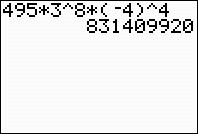Binomial Theorem
 Binomial Theorem (or Binomial Expansion Theorem)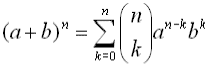Most of the syntax used in this theorem should look familiar. The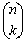notation is just another way of writing a combination such as n C (read "n choose k").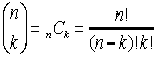The Binomial Theorem can also be written in its expanded form as: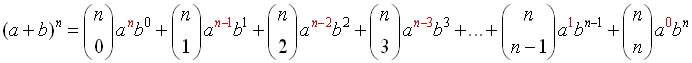Remember thatand that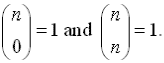Example 1:    Expand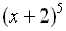.
Let a = x, b = 2, n = 5 and substitute.
(Do not substitute a value for k.)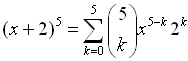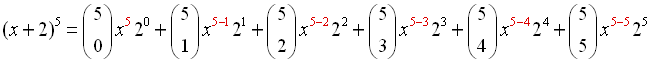Now, grab your graphing calculator to find those combination values.

Method 1:  Use the graphing calculator to evaluate the combinations on the home screen.  Remember:  Enter the top value of the combination FIRST.  Then hit MATH key, arrow right (or left) to PRB heading, and choose #3 nCr.  Now, enter the bottom value of the combination.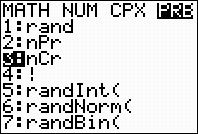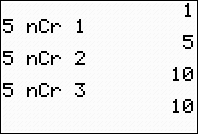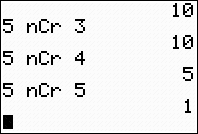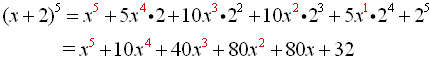Method 2: Use the graphing calculator to evaluate the combinations under the lists.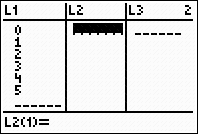In L1, enter the values 0 through the power to which the binomial is raised, in this case 5.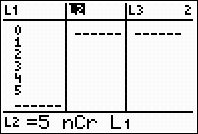In L2, enter the combination formula, using the power of the binomial as the starting value, and the entries from L1 as the ending values.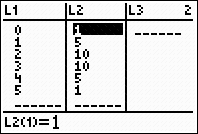The coefficients from the combinations will appear in L2.Finding a Particular Term in a Binomial Expansion

 The r th term of the expansion of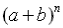is: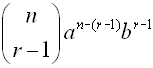Example 2:    Find the 5th term of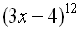.
Let r = 5, a = (3x), b = (-4),
n
= 12 and substitute.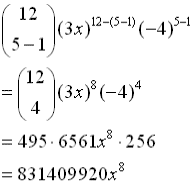Grab your calculator. Be sure to include those parentheses!(unless you do the subtraction manually)Be sure to raise each entire parentheses to the indicated powers!